# 有监督与无监督学习

• 监督学习：在这种类型的学习中，我们会得到一个带有正确标签的输入数据集，我们需要学习输入和输出之间的关系（作为函数）。手写数字分类问题是监督（分类）问题的一个例子。
• 无监督学习：在这种类型的学习中，我们几乎不知道或根本不知道我们的输出应该是什么样子。我们可以从不一定知道变量影响的数据中得出结构。在图像处理技术中，聚类就是一个例子，在这种技术中，我们不知道哪个像素属于哪个片段。

# 基于颜色量化的 K-均值聚类图像分割

import numpy as np
import matplotlib.pyplot as plt
from sklearn.cluster import KMeans
from sklearn.metrics import pairwise_distances_argmin
from sklearn.utils import shuffle
from skimage import img_as_float
from time import time

# Display the original image
plt.figure(1), plt.clf()
ax = plt.axes([0, 0, 1, 1])
plt.axis('off'), plt.title('Original image (%d colors)' %(len(np.unique(pepper)))), plt.imshow(pepper)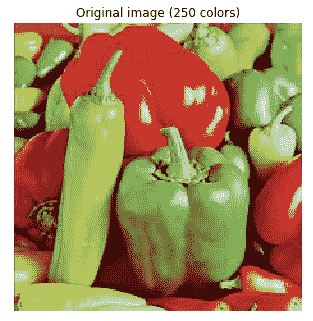n_colors = 64

# Convert to floats instead of the default 8 bits integer coding. Dividing by
# 255 is important so that plt.imshow behaves works well on float data (need to
# be in the range [0-1])
pepper = np.array(pepper, dtype=np.float64) / 255

# Load Image and transform to a 2D numpy array.
w, h, d = original_shape = tuple(pepper.shape)
assert d == 3
image_array = np.reshape(pepper, (w * h, d))

def recreate_image(codebook, labels, w, h):
"""Recreate the (compressed) image from the code book & labels"""
d = codebook.shape
image = np.zeros((w, h, d))
label_idx = 0
for i in range(w):
for j in range(h):
image[i][j] = codebook[labels[label_idx]]
label_idx += 1
return image

# Display all results, alongside original image
plt.figure(1)
plt.clf()
ax = plt.axes([0, 0, 1, 1])
plt.axis('off')
plt.title('Original image (96,615 colors)')
plt.imshow(pepper)

plt.figure(2, figsize=(10,10))
plt.clf()
i = 1
for k in [64, 32, 16, 4]:
t0 = time()
plt.subplot(2,2,i)
plt.axis('off')
image_array_sample = shuffle(image_array, random_state=0)[:1000]
kmeans = KMeans(n_clusters=k, random_state=0).fit(image_array_sample)
print("done in %0.3fs." % (time() - t0))
# Get labels for all points
print("Predicting color indices on the full image (k-means)")
t0 = time()
labels = kmeans.predict(image_array)
print("done in %0.3fs." % (time() - t0))
plt.title('Quantized image (' + str(k) + ' colors, K-Means)')
plt.imshow(recreate_image(kmeans.cluster_centers_, labels, w, h))
i += 1
plt.show()

plt.figure(3, figsize=(10,10))
plt.clf()
i = 1
for k in [64, 32, 16, 4]:
t0 = time()
plt.subplot(2,2,i)
plt.axis('off')
codebook_random = shuffle(image_array, random_state=0)[:k + 1]
print("Predicting color indices on the full image (random)")
t0 = time()
labels_random = pairwise_distances_argmin(codebook_random,
image_array,
axis=0)
print("done in %0.3fs." % (time() - t0))
plt.title('Quantized image (' + str(k) + ' colors, Random)')
plt.imshow(recreate_image(codebook_random, labels_random, w, h))
i += 1
plt.show()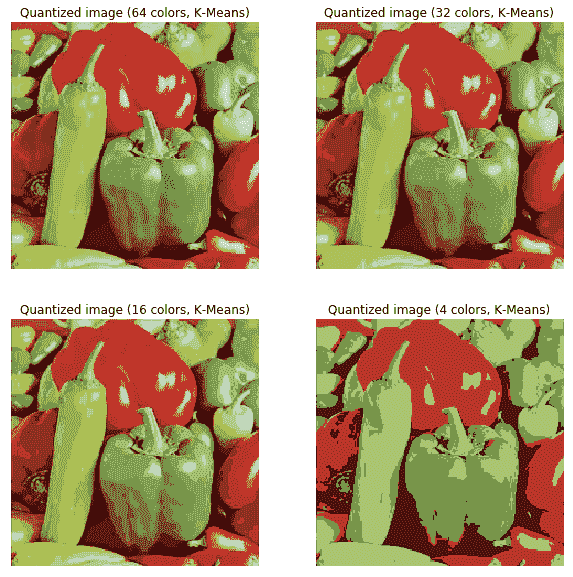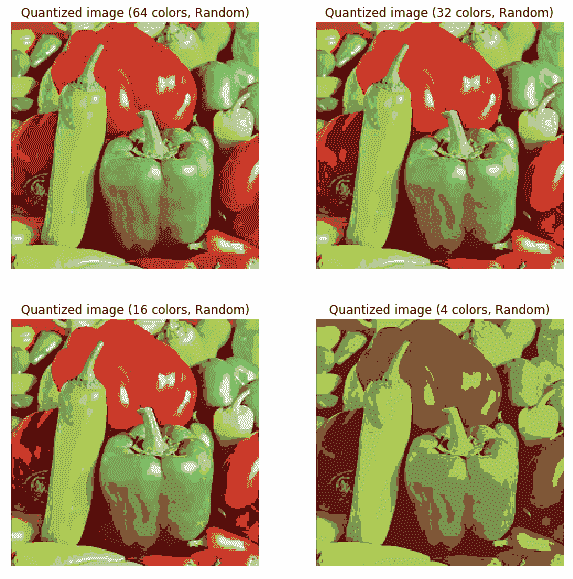# 用于图像分割的谱聚类方法

from sklearn import cluster ...


# 基于 PCA 的降维与可视化

import numpy as npimport matplotlib.pylab as plt from sklearn.datasets import load_digits from sklearn.preprocessing import StandardScalerfrom sklearn.decomposition import PCAfrom sklearn.pipeline import Pipelinedigits = load_digits() #print(digits.keys())print(digits.data.shape)j = 1np.random.seed(1)fig = plt.figure(figsize=(3,3)) fig.subplots_adjust(left=0, right=1, bottom=0, top=1, hspace=0.05, wspace=0.05) for i ...


# 二维投影与可视化

pca_digits=PCA(2)
digits.data_proj = pca_digits.fit_transform(digits.data)
print(np.sum(pca_digits.explained_variance_ratio_))
# 0.28509364823696987
plt.figure(figsize=(15,10))
plt.scatter(digits.data_proj[:, 0], digits.data_proj[:, 1], lw=0.25, c=digits.target, edgecolor='k', s=100, cmap=plt.cm.get_cmap('cubehelix', 10))
plt.xlabel('PC1', size=20), plt.ylabel('PC2', size=20), plt.title('2D Projection of handwritten digits with PCA', size=25)
plt.colorbar(ticks=range(10), label='digit value')
plt.clim(-0.5, 9.5)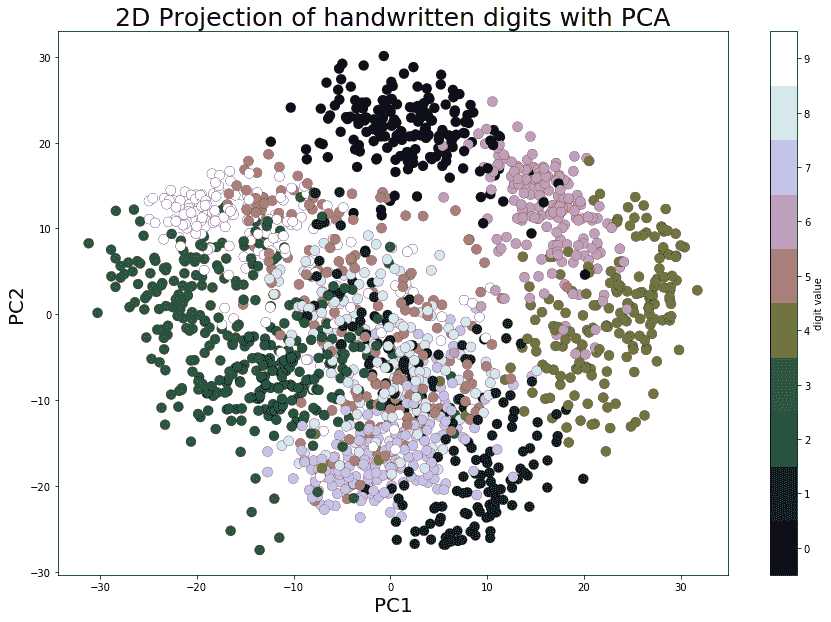# PCA 特征脸

from sklearn.datasets import fetch_olivetti_faces faces = fetch_olivetti_faces().dataprint(faces.shape) # there are 400 faces each of them is of 64x64=4096 pixelsfig = plt.figure(figsize=(5,5)) fig.subplots_adjust(left=0, right=1, bottom=0, top=1, hspace=0.05, wspace=0.05) # plot 25 random facesj = 1np.random.seed(0)for i in np.random.choice(range(faces.shape), 25):     ax = fig.add_subplot(5, 5, j, xticks=[], yticks=[])     ax.imshow(np.reshape(faces[i,:],(64,64)), cmap=plt.cm.bone, interpolation='nearest')     j += 1plt.show() ...


# 特征脸

fig = plt.figure(figsize=(5,2))
fig.subplots_adjust(left=0, right=1, bottom=0, top=1, hspace=0.05, wspace=0.05)
# plot the first 10 eigenfaces
for i in range(10):
ax = fig.add_subplot(2, 5, i+1, xticks=[], yticks=[])
ax.imshow(np.reshape(pipeline.named_steps['pca'].components_[i,:], (64,64)), cmap=plt.cm.bone, interpolation='nearest')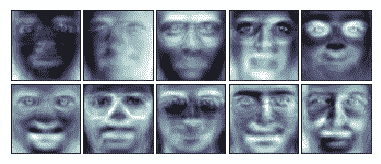# 重建

# face reconstructionfaces_inv_proj = pipeline.named_steps['pca'].inverse_transform(faces_proj) #reshaping as 400 images of 64x64 dimension fig = plt.figure(figsize=(5,5)) fig.subplots_adjust(left=0, right=1, bottom=0, top=1, hspace=0.05, wspace=0.05) # plot the faces, each image is 64 by 64 dimension but 8x8 pixels j = 1np.random.seed(0)for i in np.random.choice(range(faces.shape), 25):

 ax = fig.add_subplot(5, ...


# 特征分解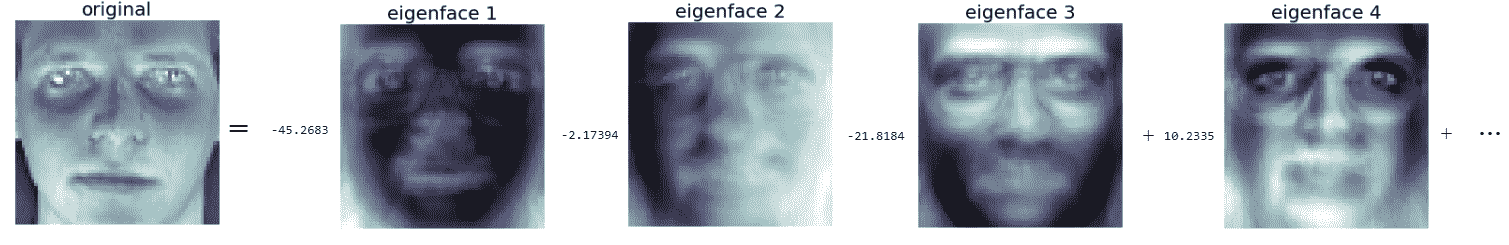# 下载 MNIST（手写数字）数据集

# Function that downloads a specified MNIST data file from Yann Le Cun's website
urlretrieve(source + filename, filename)

if not os.path.exists(filename):
with gzip.open(filename, 'rb') as f:
data = data.reshape(-1,784)
return data

if not os.path.exists(filename):
with gzip.open(filename, 'rb') as f:
return data


test_labels = load_mnist_labels('t10k-labels-idx1-ubyte.gz')

print(train_data.shape)
# (60000, 784)         ## 60k 28x28 handwritten digits
print(test_data.shape)
# (10000, 784)         ## 10k 2bx28 handwritten digits


# 可视化数据集

## Define a function that displays a digit given its vector representationdef show_digit(x, label): pylab.axis('off') pylab.imshow(x.reshape((28,28)), cmap=pylab.cm.gray) pylab.title('Label ' + str(label)) pylab.figure(figsize=(10,10))for i in range(25): pylab.subplot(5, 5, i+1) show_digit(test_data[i,], test_labels[i])pylab.tight_layout()pylab.show()


# 训练 kNN、高斯贝叶斯和 SVM 模型对 MNIST 进行分类

• K 近邻
• 高斯贝叶斯分类器（生成模型）
• 支持向量机SVM分类器

# 欧氏距离的平方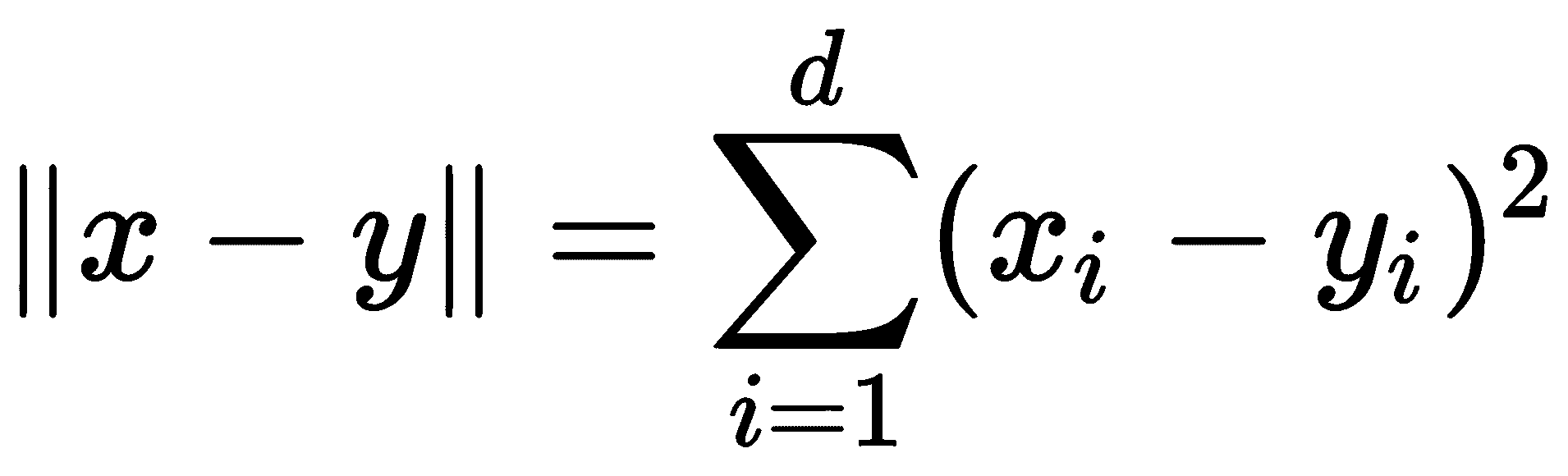# 计算最近邻

k-最近邻的简单实现将扫描每个测试图像的每个训练图像。以这种方式执行最近邻分类将需要完全通过训练集才能对单个点进行分类。如果Rd中有N个训练点，这需要O（Nd）时间，这将非常缓慢。幸运的是，如果我们愿意花一些时间预处理训练集，有更快的方法来执行最近邻查找。scikit 学习库快速实现了两种有用的最近邻数据结构：ball 树和 k-d 树。下面的代码演示如何在训练时创建 ball 树数据结构，然后将其用于。。。

# 评估分类器的性能

# evaluate the classifier
t_accuracy = sum(test_predictions == test_labels) / float(len(test_labels))
t_accuracy
# 0.96909999999999996

import pandas as pd
import seaborn as sn
from sklearn import metrics

cm = metrics.confusion_matrix(test_labels,test_predictions)
df_cm = pd.DataFrame(cm, range(10), range(10))
sn.set(font_scale=1.2)#for label size
sn.heatmap(df_cm, annot=True,annot_kws={"size": 16}, fmt="g")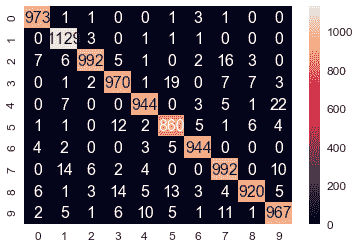• 成功案例1-NN 预测标签=真标签=0
• 故障案例1-NN 预测标签=2，真标签=3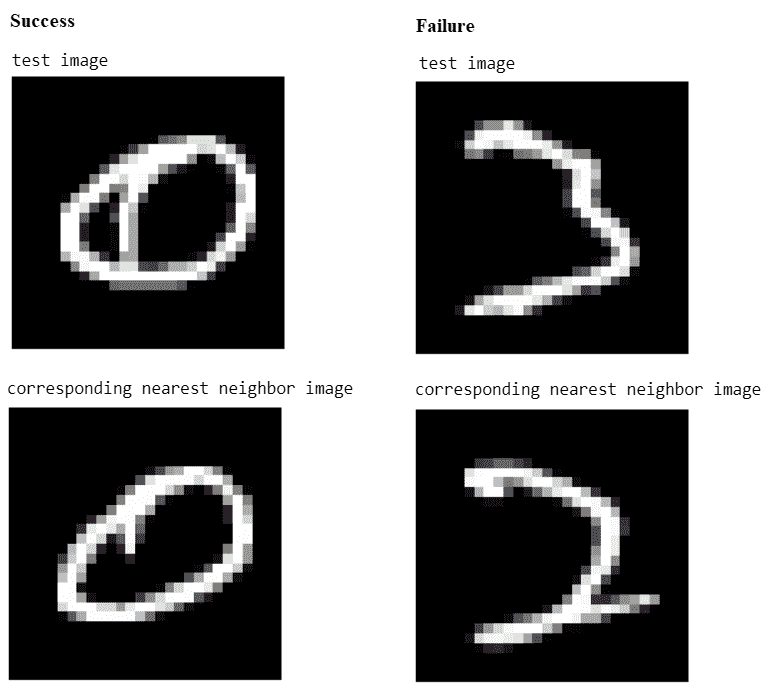# 培训生成模型-计算高斯参数的最大似然估计

• πj：标签的频率（即之前的频率）
• μj：784 维平均向量
• j：784 x 784 协方差矩阵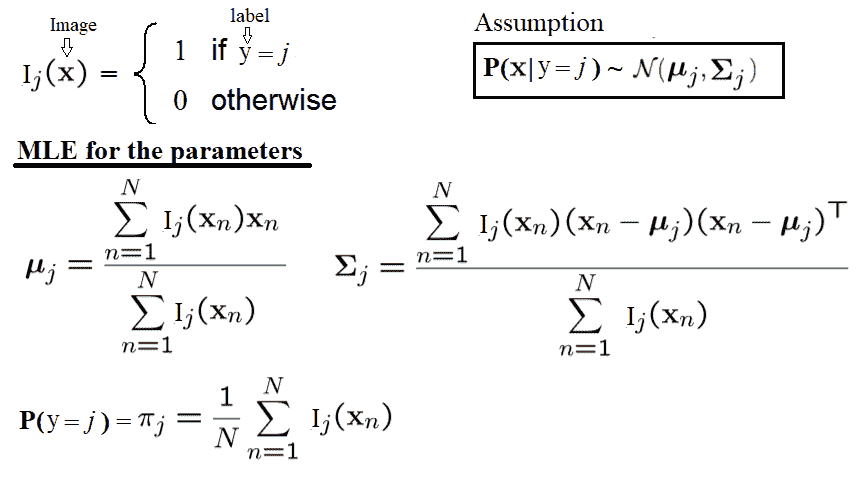def display_char(image):
plt.imshow(np.reshape(image, (28,28)), cmap=plt.cm.gray)
plt.axis('off')
plt.show()

def fit_generative_model(x,y):
k = 10 # labels 0,1,...,k-1
d = (x.shape) # number of features
mu = np.zeros((k,d))
sigma = np.zeros((k,d,d))
pi = np.zeros(k)
c = 3500 #10000 #1000 #100 #10 #0.1 #1e9
for label in range(k):
indices = (y == label)
pi[label] = sum(indices) / float(len(y))
mu[label] = np.mean(x[indices,:], axis=0)
sigma[label] = np.cov(x[indices,:], rowvar=0, bias=1) + c*np.eye(d)
return mu, sigma, pi

mu, sigma, pi = fit_generative_model(train_data, train_labels)
display_char(mu)
display_char(mu)
display_char(mu)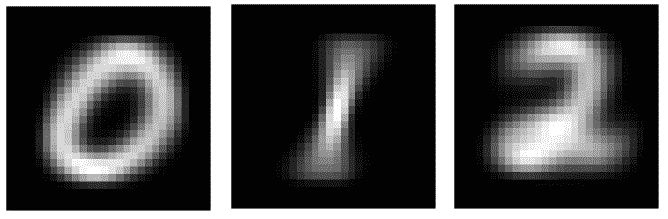# 计算后验概率对试验数据和模型评估进行预测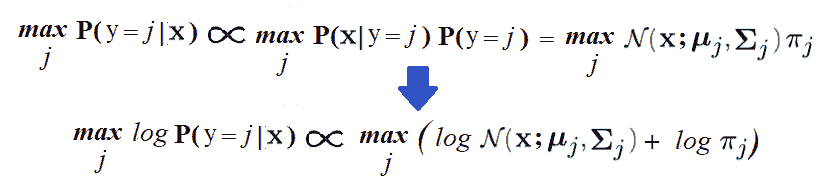# Compute log Pr(label|image) for each [test image,label] pair.k = 10score ...


# 分类器

SVM 是一种非常复杂的二元分类器，它使用二次规划来最大化分离超平面之间的边界。利用 1-vs-all 或 1-vs-1 技术，将二元 SVM 分类器扩展到处理多类分类问题。我们将使用 scikit learn 的实现SVC()，使用多项式核（2 次）拟合（训练）训练数据集的软边界（核化）SVM 分类器，然后使用score()函数预测测试图像的标签。

from sklearn.svm import SVC
clf = SVC(C=1, kernel='poly', degree=2)
clf.fit(train_data,train_labels)
print(clf.score(test_data,test_labels))
# 0.9806
test_predictions = clf.predict(test_data)
cm = metrics.confusion_matrix(test_labels,test_predictions)
df_cm = pd.DataFrame(cm, range(10), range(10))
sn.set(font_scale=1.2)
sn.heatmap(df_cm, annot=True,annot_kws={"size": 16}, fmt="g")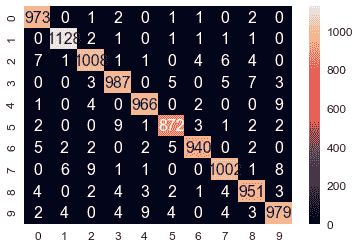wrong_indices = test_predictions != test_labels
wrong_digits, wrong_preds, correct_labs = test_data[wrong_indices], test_predictions[wrong_indices], test_labels[wrong_indices]
print(len(wrong_pred))
# 194
pylab.title('predicted: ' + str(wrong_preds) + ', actual: ' + str(correct_labs))
display_char(wrong_digits)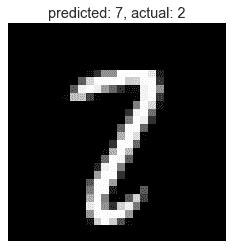# 基于 Haar-like 特征的人脸检测和 AdaBoost-Viola-Jones 级联分类器

Viola-Jones 算法通常使用基本面片大小（例如，24 x 24 像素），它在图像上来回滑动，并计算大量类似 Haar 的特征（24 x 24 面片的 160000 个可能特征，尽管通过适当选择 6000 个特征子集，它们已达到 95%的准确率）；这些功能是可以提取的，但要在每个补丁上运行 6000 个功能需要付出很大的努力。因此，一旦确定并组装了这些特性，就会构建一些快速拒绝器。这是基于这样的想法，即在一张完整的图像中，我们检查的大多数可能位置都不会包含人脸，因此，总体而言，快速拒绝比在其上投入太多更快。如果该位置不包含面，我们将丢弃它并继续，然后再进行更多的计算检查。这是 Viola Jones 实现其性能的另一个技巧，如下图所示：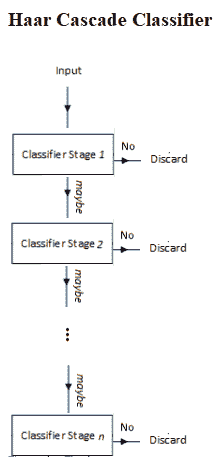Haar-like 功能的一个很好的优点是计算速度非常快（由于积分图像技术），这导致了 Viola-Jones 性能的最后一个方面。基本面片本身可以缩放，最终级联中的特征可以非常快速地评估，以搜索不同大小的对象，而无需图像金字塔。综合起来，Viola-Jones 培训工作流程如下图所示：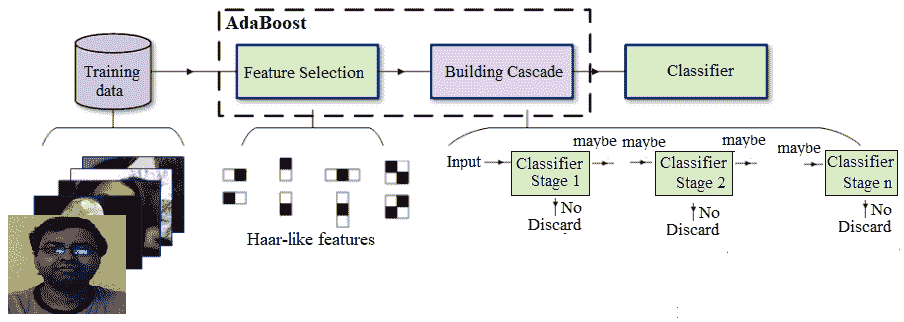# 基于类 Haar 特征描述子的人脸分类

Viola Jones 使用类似 Haar 的特征描述符实现了第一个实时人脸检测器。以下示例取自 scikit 图像的示例，该示例演示了提取、选择和分类类 Haar 特征，以检测人脸与非人脸。显然，这是一个二值分类问题。

# 用随机森林集成分类器寻找人脸分类中最重要的类 Haar 特征

# For speed, only extract the two first types of features
feature_types = ['type-2-x', 'type-2-y']
# Build a computation graph using dask. This allows using multiple CPUs for
# the computation step
X = delayed(extract_feature_image(img, feature_types)
for img in images)
# Compute the result using the "processes" dask backend
t_start = time()
X = np.array(X.compute(scheduler='processes'))
time_full_feature_comp = time() - t_start
y = np.array( * 100 +  * 100)
X_train, X_test, y_train, y_test = train_test_split(X, y, train_size=150, random_state=0, stratify=y)
print(time_full_feature_comp)
# 104.87986302375793
print(X.shape, X_train.shape)
# (200, 101088) (150, 101088)

from sklearn.metrics import roc_curve, auc, roc_auc_score
# Extract all possible features to be able to select the most salient.
feature_coord, feature_type = \
haar_like_feature_coord(width=images.shape, height=images.shape,
feature_type=feature_types)

# Train a random forest classifier and check performance
clf = RandomForestClassifier(n_estimators=1000, max_depth=None,
max_features=100, n_jobs=-1, random_state=0)
t_start = time()
clf.fit(X_train, y_train)
time_full_train = time() - t_start
print(time_full_train)
# 1.6583366394042969
auc_full_features = roc_auc_score(y_test, clf.predict_proba(X_test)[:, 1])
print(auc_full_features)
# 1.0

# Sort features in order of importance, plot six most significant
idx_sorted = np.argsort(clf.feature_importances_)[::-1]

fig, axes = pylab.subplots(5, 5, figsize=(10,10))
for idx, ax in enumerate(axes.ravel()):
image = images
image = draw_haar_like_feature(image, 0, 0, images.shape, images.shape,
[feature_coord[idx_sorted[idx]]])
ax.imshow(image), ax.set_xticks([]), ax.set_yticks([])
fig.suptitle('The most important features', size=30)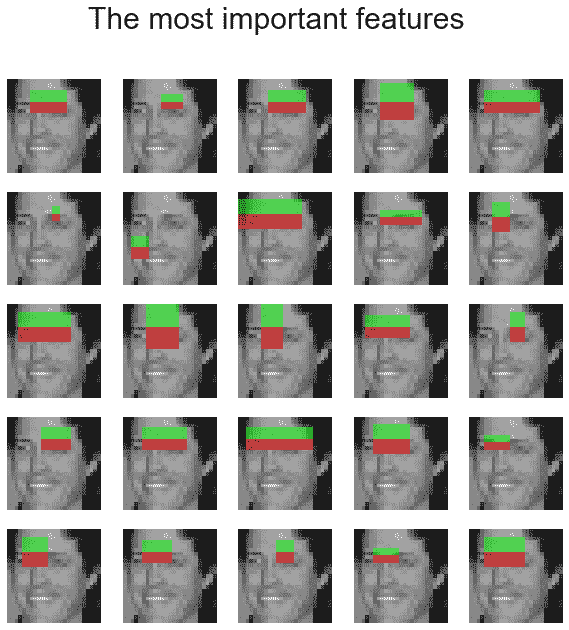# 养猪

SVM 训练器选择最佳超平面，以从训练集中分离正示例和负示例。将这些块描述符串联起来，转换为 SVM 训练器的输入格式，并适当标记为正或负。训练器通常输出一组支持向量，即，训练集中最能描述超平面的示例。超平面是区分正例和负例的学习决策边界。SVM 模型随后使用这些支持向量对测试图像中的 HOG 描述符块进行分类，以检测对象的存在/不存在。

# 用 HOG-SVM 计算包围盒

import numpy as np
import cv2
import matplotlib.pylab as pylab
# create HOG descriptor using default people (pedestrian) detector
hog = cv2.HOGDescriptor()
hog.setSVMDetector(cv2.HOGDescriptor_getDefaultPeopleDetector())
# run detection, using a spatial stride of 4 pixels (horizontal and vertical), a scale stride of 1.02, and # zero grouping of rectangles (to demonstrate that HOG will detect at potentially multiple places in the
# scale pyramid)
(foundBoundingBoxes, weights) = hog.detectMultiScale(img, winStride=(4, 4), padding=(8, 8), scale=1.02, finalThreshold=0)
print(len(foundBoundingBoxes)) # number of boundingboxes
# 357
# copy the original image to draw bounding boxes on it for now, as we'll use it again later
imgWithRawBboxes = img.copy()
for (hx, hy, hw, hh) in foundBoundingBoxes:
cv2.rectangle(imgWithRawBboxes, (hx, hy), (hx + hw, hy + hh), (0, 0, 255), 1)
pylab.figure(figsize=(20, 12))
imgWithRawBboxes = cv2.cvtColor(imgWithRawBboxes, cv2.COLOR_BGR2RGB)
pylab.imshow(imgWithRawBboxes, aspect='auto'), pylab.axis('off'), pylab.show()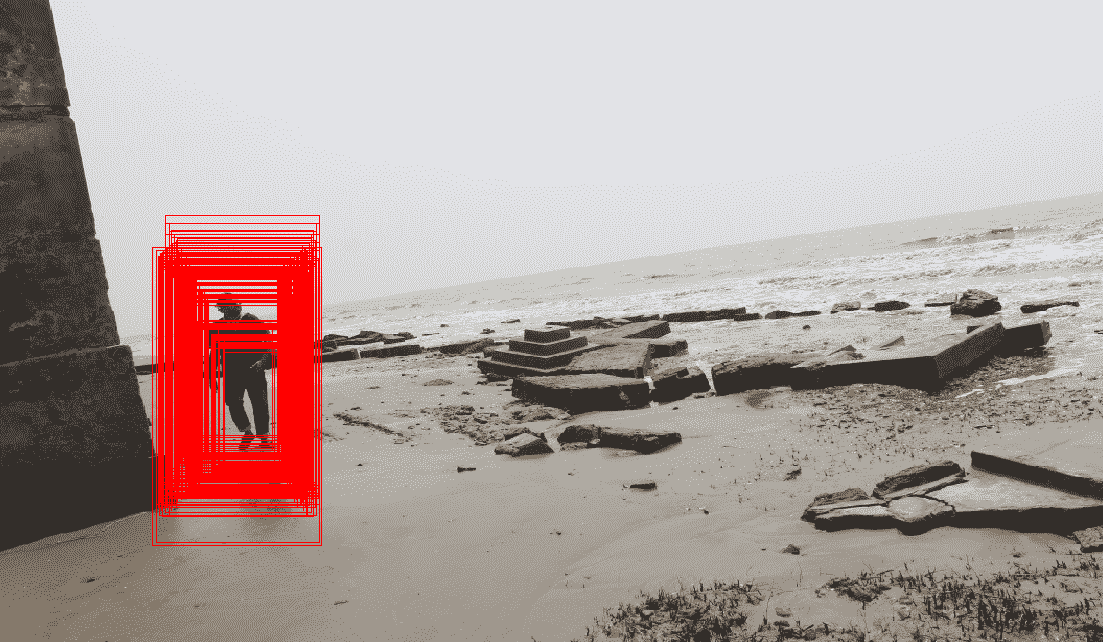# 非最大值抑制

from imutils.object_detection import non_max_suppression# convert our bounding boxes from format (x1, y1, w, h) to (x1, y1, x2, y2)rects = np.array([[x, y, x + w, y + h] for (x, y, w, h) in foundBoundingBoxes])# run non-max suppression on these based on an overlay op 65%nmsBoundingBoxes = non_max_suppression(rects, probs=None, overlapThresh=0.65)print(len(rects), len(nmsBoundingBoxes))# 357 1# draw the final bounding boxesfor (x1, y1, x2, y2) in nmsBoundingBoxes: cv2.rectangle(img, (x1, y1), (x2, y2), (0, 255, 0), ...


# 问题

1. 使用 k 均值聚类对图像进行阈值化（使用number of clusters=2），并将结果与大津的结果进行比较。
2. 使用 scikit learn 的cluster.MeanShift()mixture.GaussianMixture()函数，分别使用 mean shift 和 GMM-EM 聚类方法对图像进行分割，这是另外两种流行的聚类算法。
3. 使用 Isomap（从sklearn.manifold开始）进行非线性降维，并可视化二维投影。它是否比 PCA 的线性降维更好？用 TSNE 重复练习（再次从sklearn.manifold开始）。
4. 编写一个 Python 程序来显示几个主要特征面的加权线性组合确实近似于一个面。
5. 表明特征脸也可以用于原始人脸检测（和识别）。。。